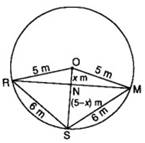No products in the cart.

# Three girls Reshma, Salma and Mandip …## CBSE, JEE, NEET, CUET

Question Bank, Mock Tests, Exam Papers

NCERT Solutions, Sample Papers, Notes, Videos

Three girls Reshma, Salma and Mandip are playing a game by standing on a circle of radius 5m drawn in a park. Reshma throws a ball to Salma, Salma to Mandip, Mandip to Reshma. If the distance between Reshma and Salma and between Salma and Mandip is 6m each, what is the distance between Reshma and Mandip? Please give answer with Given, To Prove and Construction

Preeti Dabral 5 months, 3 weeks agoIn {tex}\Delta \mathrm { NOR }{/tex} and {tex}\Delta \mathrm { NOM }{/tex}
ON = ON |Common
{tex}\angle \mathrm { NOR } = \angle \mathrm { NOM }{/tex} {tex}| \because{/tex} Equal chords of a circle subtend equal
angle at the centre
OR = OM |Radii of a circle
{tex}\therefore \triangle \mathrm { NOR } \cong \Delta \mathrm { NOM }{/tex} [SAS Rule]
{tex}\therefore \angle O N R = \angle O N M{/tex} [c.p.c.t]
and NR = NM [c.p.c.t.]
But {tex}\angle O N R + \angle O N M = 180 ^ { \circ }{/tex} |Linear Pair Axiom
{tex}\therefore \angle O \mathrm { NR } = \angle \mathrm { O } \mathrm { NM } = 90 ^ { \circ }{/tex}
{tex}\triangle{/tex} ON is the perpendicular bisector of RM,
Draw bisector SN of {tex}\angle \mathrm { R } \mathrm { SM }{/tex} to intersect the chord RM in N.
In {tex}\Delta \mathrm { RSN }{/tex} and {tex}\Delta \mathrm { MSN }{/tex}
RS = MS (= 6 cm each)
SN = SN [Common]
{tex}\angle R S N = \angle M S N{/tex} [By construction]
{tex}\therefore \Delta R S N \cong \Delta \mathrm { NSN }{/tex} [SAS Rule]
{tex}\therefore \angle R N S = \angle M N S{/tex} [c.p.c.t]
and RN = MN [c.p.c.t]
But {tex}\angle \mathrm { RNS } + \angle \mathrm { MNS } = 180 ^ { \circ }{/tex} |Linear Pair Axion
{tex}\therefore \angle R N S = \angle M N S = 90 ^ { \circ }{/tex}
{tex}\therefore \mathrm { SN }{/tex} is the perpendicular bisector of RM and therefore passes through O when produced.
Let ON = x m
Then SN = (5 - x) m
In right triangle ONR,
x2 + RN2 = 52, ------ (1) |By Pythagoras theorem
In right triangle SNR,
(5-x)2 + RN2 = 62 ---- (2) |By Pythagoras theorem
From (1),
RN2 = 52 - x2
From (2),
RN2 = 62 - (5 - x)2
Equating the two values of RN2, we get
52 - x2 = 62 - (5 -x)2
{tex}\Rightarrow 25 - x ^ { 2 } = 36 - ( 25 - 10 x + x ) ^ { 2 }{/tex} {tex}\Rightarrow 25 - x ^ { 2 } = 36 - 25 + 10 x - x ^ { 2 }{/tex}
{tex}\Rightarrow 25 - x ^ { 2 } = 11 + 10 x - x ^ { 2 }{/tex}{tex}\Rightarrow 25 - 11 = 10 x{/tex}
{tex}\Rightarrow{/tex} 14 = 10 x {tex}\Rightarrow{/tex}10x = 14
{tex}\Rightarrow x = \frac { 14 } { 10 } = 1.4{/tex}
Putting x = 1.4 in (1), we get
(1.4)2 + RN2 = 52
{tex}\Rightarrow R N ^ { 2 } = 5 ^ { 2 } - ( 1.4 ) ^ { 2 }{/tex} {tex}\Rightarrow \mathrm { RN } ^ { 2 } = 25 - 1.96{/tex}
{tex}\Rightarrow \mathrm { RN } ^ { 2 } = 23.04 \Rightarrow \mathrm { RN } = \sqrt { 23.04 }{/tex}
{tex}\Rightarrow{/tex} RN = 4.8
{tex}\therefore{/tex} RM = 2 RN = {tex}2 \times 4.8 \mathrm { m } = 9.6 \mathrm { m }{/tex}
Hence, the distance between Reshma and Mandip is 9.6 m.

Related Questions## myCBSEguide

Trusted by 1 Crore+ Students## Test Generator

Create papers online. It's FREE.## CUET Mock Tests

75,000+ questions to practice only on myCBSEguide app